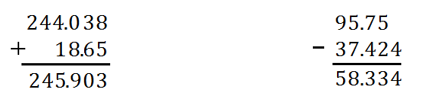# Multi-Digit and Fraction Computation

## Objective

Add and subtract decimals using the standard algorithm.

## Common Core Standards

### Core Standards

?

• 6.NS.B.3 — Fluently add, subtract, multiply, and divide multi-digit decimals using the standard algorithm for each operation.

?

• 5.NBT.B.7

## Criteria for Success

?

1. Understand the role that place value plays in addition and subtraction of decimals.
2. Accurately use the standard algorithm to add and subtract decimals.
3. Use addition and subtraction to solve decimal word problems.

## Tips for Teachers

?

• In fifth grade, students computed with decimals using all four operations and various strategies. In sixth grade, adding and subtracting with decimals is a fluency expectation. Use the problems in this lesson to review any strategies from fifth grade as needed so that students have a conceptual understanding to support the standard algorithm. Ensure students have opportunities to build towards fluency throughout the unit and the year. See our Guide to Procedural Skill and Fluency for additional information and strategies.
• The following tools or materials are useful for this lesson: large graph or grid paper for computations, calculators to check answers.

#### Fishtank Plus

• Problem Set
• Student Handout Editor
• Vocabulary Package

## Anchor Problems

?

### Problem 1

Jayden has $20.56. He buys an apple for 79 cents and a granola bar for$1.76.

1. How much money did Jayden spend?
2. How much money does Jayden have now?

#### References

Illustrative Mathematics Jayden's Snacks

Jayden's Snacks, accessed on Sept. 28, 2017, 2:25 p.m., is licensed by Illustrative Mathematics under either the CC BY 4.0 or CC BY-NC-SA 4.0. For further information, contact Illustrative Mathematics.

### Problem 2

Dan is hiking along the Pacific Coast Trail. On Sunday morning, he hikes 5.83 miles before stopping for lunch. At the end of the day, Dan has hiked a total of 12.4 miles. How many miles did he hike after lunch?

### Problem 3

The two problems below have been solved incorrectly. Explain the error in each problem and then correctly solve each one.

 ${ 244.038+18.65 }$ ${95.75-37.424}$## Problem Set

?The following resources include problems and activities aligned to the objective of the lesson that can be used to create your own problem set.

• Include fluency practice with adding and subtracting decimals.
• Include error analysis problems of the standard algorithm applied incorrectly.